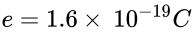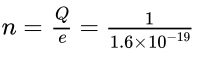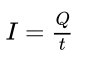# Charge → (q)

• A charge is a characteristic unit of the matter by means of which matter experiences electric forces. It can be either negative (electron) or positive (proton).
• “Coulomb” is the SI unit of charge, represented by C.

# Net charge (Q) – Total charge

A 1C Net charge is equivalent to the charge contained in nearly electrons 6×1018 electrons.

Q = ne (n is no. of electrons)(negative charge on an electron)# Current (I)

• The rate of flow of net charge is called current. Denoted by (I)• Electric current can also be defined as the amount of charge flowing through a unit cross-section per second.
• SI unit of current is “Ampere”. Denoted by A.

Ampere → Defined as one coulomb of charge flowing per second.In an electric circuit the electric current flow in an opposite direction to the flow of electron (–ve charge). This current is called conventional current. It flows from the +ve terminal battery or cell to –ve terminal. A small quantity of current is expressed in

mA (milliAmpere) = 10-3 A

µA (microAmpere) = 10-6 A